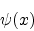Next: Problems Up: Schrodinger's equation Previous: Stationary states

#### Solution of Schrodinger equation

The time independent Schrodinger equation contains two unknowns, the energy E and the wave function.

In order to solve the equation additional boundary conditions are invoked which are specific to the problem in question.

Specific problems have been discussed in detail in all the text books on Quantum Mechanics and would be discussed here in future. Exact solution of the Schrodinger equation can be obtained and have been discussed for a particle in a 1-dimensional infinitely deep potential well, particle in a 3-dimensional box, the linear harmonic oscillator, transmission of a free particle through a barrier and the Hydrogen atom.

A couple of representative problems are given to test the understanding after going through the relevant portion of the text books.

Subsections

Abhijit Poddar
2007-09-27